# ELEN 280 Final Exam Winter 2017 .pdf

### File information

Author: Michael Mcelfresh

This PDF 1.5 document has been generated by MicrosoftÂ® Word 2016, and has been sent on pdf-archive.com on 23/03/2017 at 20:56, from IP address 129.210.x.x. The current document download page has been viewed 1071 times.
File size: 809.44 KB (6 pages).
Privacy: public file

### Document preview

ELEN 280 – Final Exam (Winter 2017)
T/F Questions
1. YOLO
2. F : Wind turbines become cost effective as size is increased
3. T: Storage is the only solution available for integration of la ….renewable energy.
4. T: A Wind turbine extracts kinetic energy for electricity not potential
5. T: With continued improvements a single-stage heat engine…able to exceed the efficiencies
of the best fuel cells today…
6. F: The price of electricity from centralized power plants …price of fuel and is independent of
the capacity factor.
7. T: A carbon tax can help determine if one power generation…more economical than another
thereby changing finan….
8. T: Combined cycle systems generally use a gas turbine to..generator directly and to then
recover turbine exhaust…steam generation cycle.
9. F: CHP systems are limited to natural gas burning turbine….
10. T: Heat driven air-conditioning (e.g. absorption chilled..) are the latest technological
breakthrough in AC and …

Fill in the Blanks:
1. Systems where the heat is recoevered when producing electricity are WASTE HEAT
RECOVERING UNIT system.
2. NIH is an acronym for NOT INVENTED HERE
3. A carbon tax is one method for providing incentives for people to…carbon. A second
method for reducing carbon production, that has launched in California, is CAP AND
4. DUCK CURVE refers to the curve that is used …impacts of high levels of solar PV on the
grid.
5. PLANNED OBSOLESCENCE was coined by …describe designing for a known time for
failure.

QUESTION 1
Use the spreadsheet to calculate the U, UA, total UA and Thermal Index of a house, given a
spreadsheet with R Values and Area of each surface. He notes UA(inf) = 0.0018 nV (BTU-hr-F).
Use infiltration to add to the total UA value.

QUESTION 2

2b. Determine the learning coefficient for the curve in part a
0.82 = 2^(-b)
- b * log 2 = log 0.82
-b = -0.286

b = 0.286

QUESTION 3
1. Sizing a Solar PV System
The Rusk’s own fairly large house near San Francisco and the following spreadsheet shows their monthto-month energy use for two years.

1a. What is
average energy use? Why do you think their use peaks twice a year?
𝐀𝐧𝐬) 𝐌𝐨𝐧𝐭𝐡𝐥𝐲 𝐩𝐨𝐰𝐞𝐫 𝐚𝐯𝐞𝐫𝐚𝐠𝐞 =

their monthly

𝟒𝟑. 𝟗 𝐤𝐖𝐡/𝐝𝐚𝐲 ∗ 𝟑𝟎 𝐝𝐚𝐲
= 𝟓𝟑. 𝟖𝟕 𝐤𝐖
𝟐𝟒 𝐡𝐨𝐮𝐫𝐬

Use peaks twice a year because the house requires more cooling in the hot summer and more
heating in the cold winter.

1b. Calculate the size of the solar PV system (kWh DC,STC) they will need in order to have a zero dollar
electricity bill annually (use an average over the two years). Assume that you are using 14.9% efficient
panels with an 80% de-rating factor. Also assume that your inverter is 95% efficient. Use an average
solar insolation of 5.5kWh/m2/day for their location.
Ans) Since the average kWh per day=43.9 kWh
The annual kWh=43.9*365=16023.5 kWh/yr
𝐏𝐃𝐂,𝐒𝐓𝐂 =

𝟏𝟔𝟎𝟐𝟑. 𝟓 𝐤𝐖𝐡/𝐲𝐫
= 𝟏𝟎. 𝟓 𝐤𝐖
𝟎. 𝟖 ∗ 𝟓. 𝟓 𝐡𝐫/𝐝𝐚𝐲 ∗ 𝟑𝟔𝟓 𝐝𝐚𝐲/𝐲𝐫 ∗ 𝟎. 𝟗𝟓

1c. Assume this system will be mounted on the roof. How big will the area of solar collector be? Is this
a reasonable size for a house (i.e. are roofs actually this big)?
𝐀𝐧𝐬) 𝐀 =

𝐏𝐃𝐂,𝐒𝐓𝐂
𝟏𝟎. 𝟓
=
= 𝟕𝟎. 𝟒 𝐦𝟐
𝛈
𝟎. 𝟏𝟒𝟗

No it is not reasonable size for a house in the United States. South-facing areas are usually less than
𝟕𝟎. 𝟒 𝐦𝟐

1d. What is the AC,PTC per square meter for the system? Assume that the 80% de-rating factor gets you
from DC,STC to DC,PTC.
Ans) (AC,PTC)=0.95 (DC,PTC)
(DC,PTC)=0.8 (DC,STC)
 (AC,PTC)= 0.95 * 0.8 (DC,STC)= 0.95 * 0.8 * 10.5 k= 7.98 kW

QUESTION 4

Compare the maximum amount of energy that can be recovered from the sun in form of biodiesel
versus electricity in one average year on one acre of land. Assume that values in the table are for biofuel
that can be produced in one year.

Ans:
1 gallon of diesel produces = 139000 BTUs
1 gallon of diesel produces = 139000 BTU / 3412 BTU/kWh = 40.738 kWh
Source
Yield (kWh)
Corn
611.07 – 814.76
Soybean
1629.52 – 2036.92
Safflower
3259.04 – 3666.42
Sunflower
4073.8 – 4481.18
Rapeseed
4481.18 – 5295.94
Palm Oil
25461.25 – 26479.7
Microalgae
203690 – 611070
**Multiple AC/gal with 40.738 to obtain these values.
Solar Yield:
Solar Insolation: 5.5 kWh/ (m^2 -day)
Meter squared for 1 acre = 4046.86 m^2
Days in year = 365 days
Solar Yield: 5.5 x 4046.86 x 365 = 8,124,071.45 kWh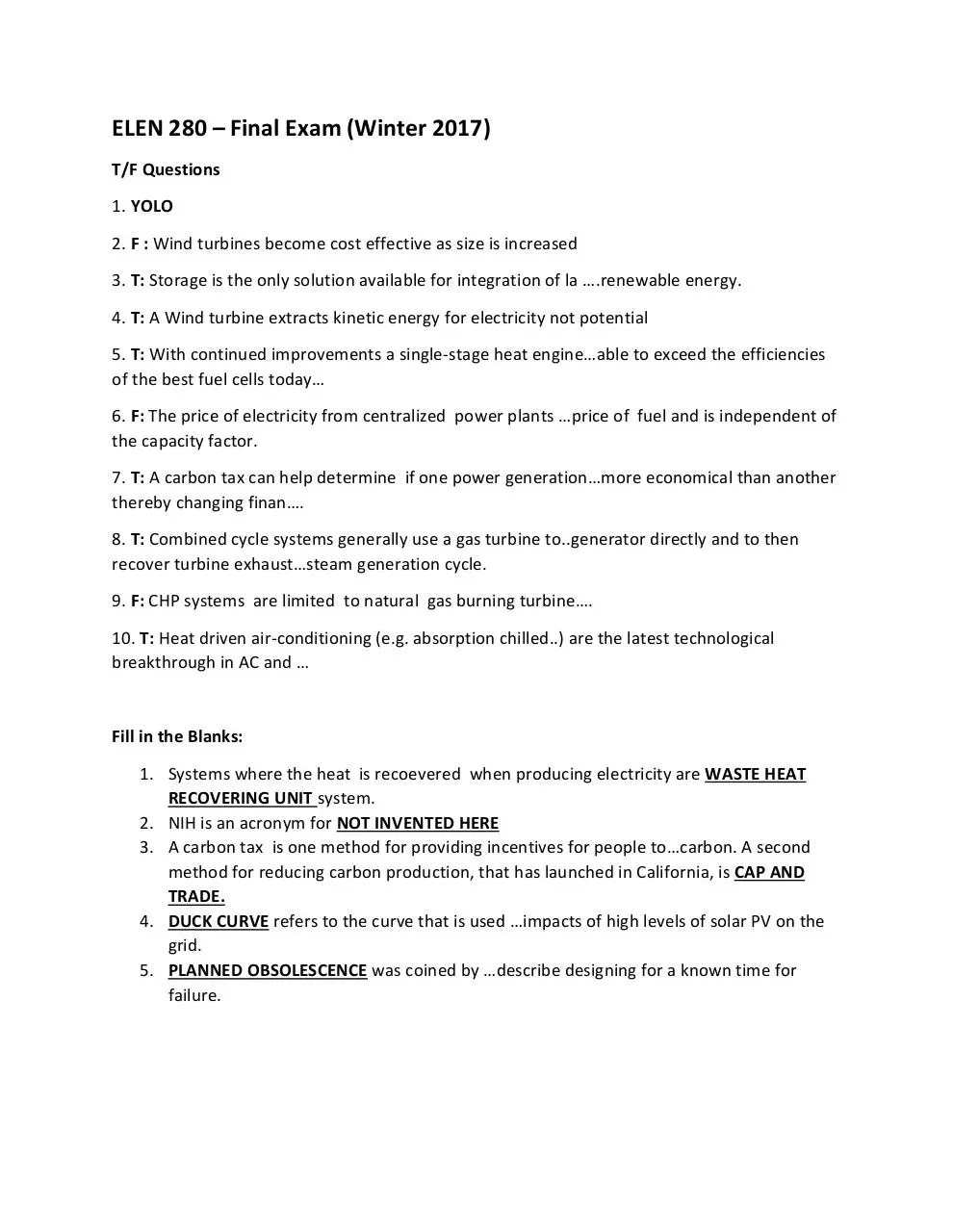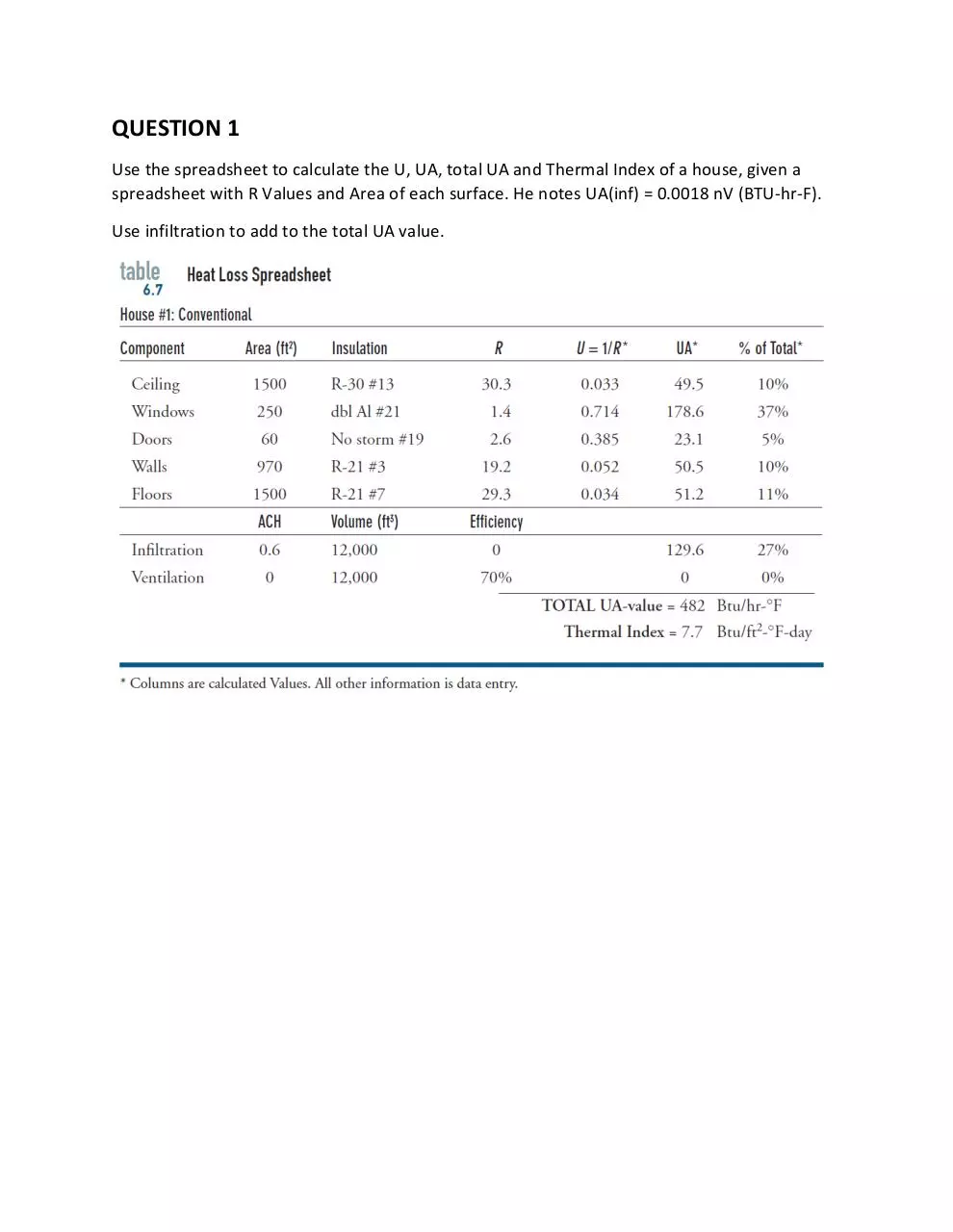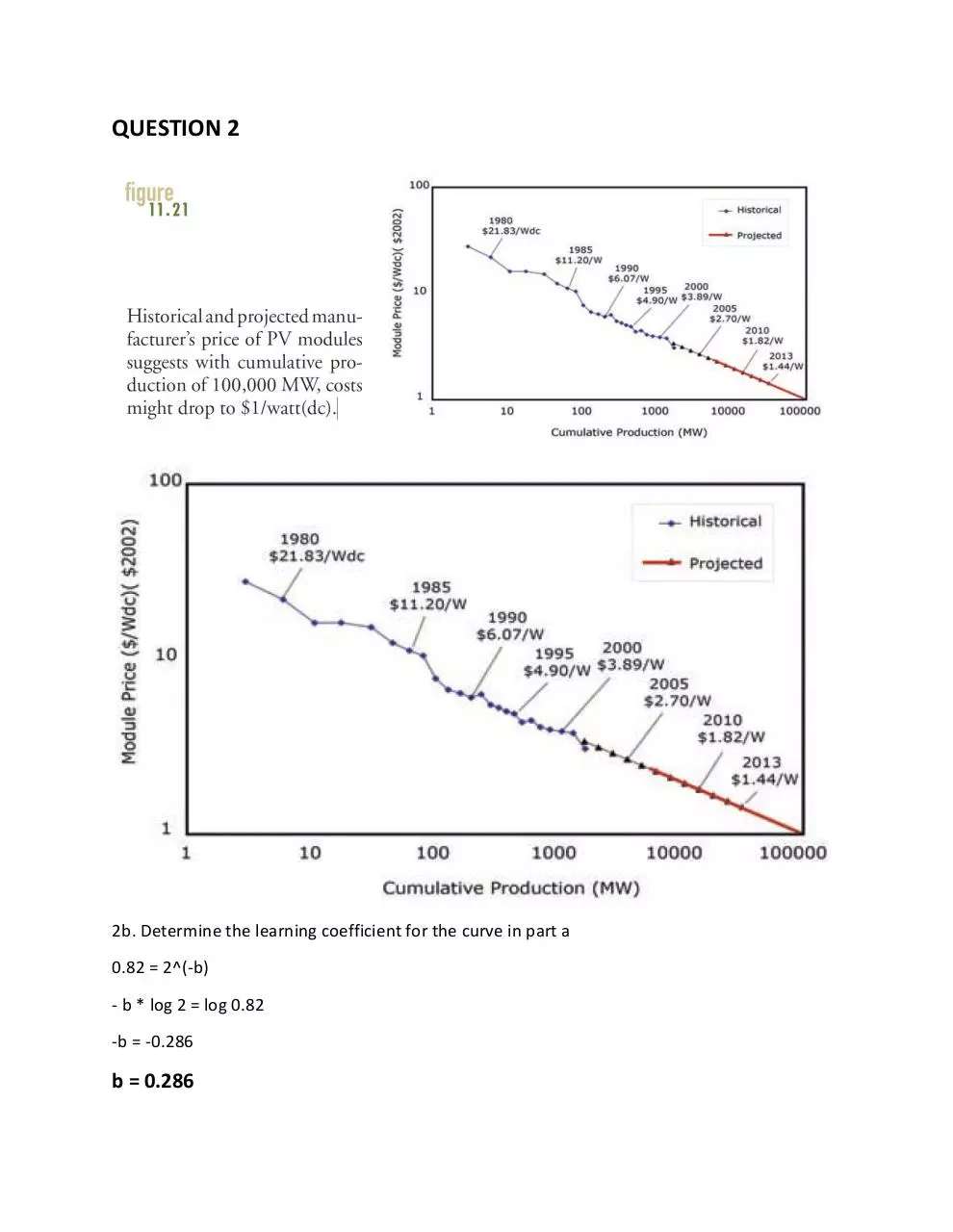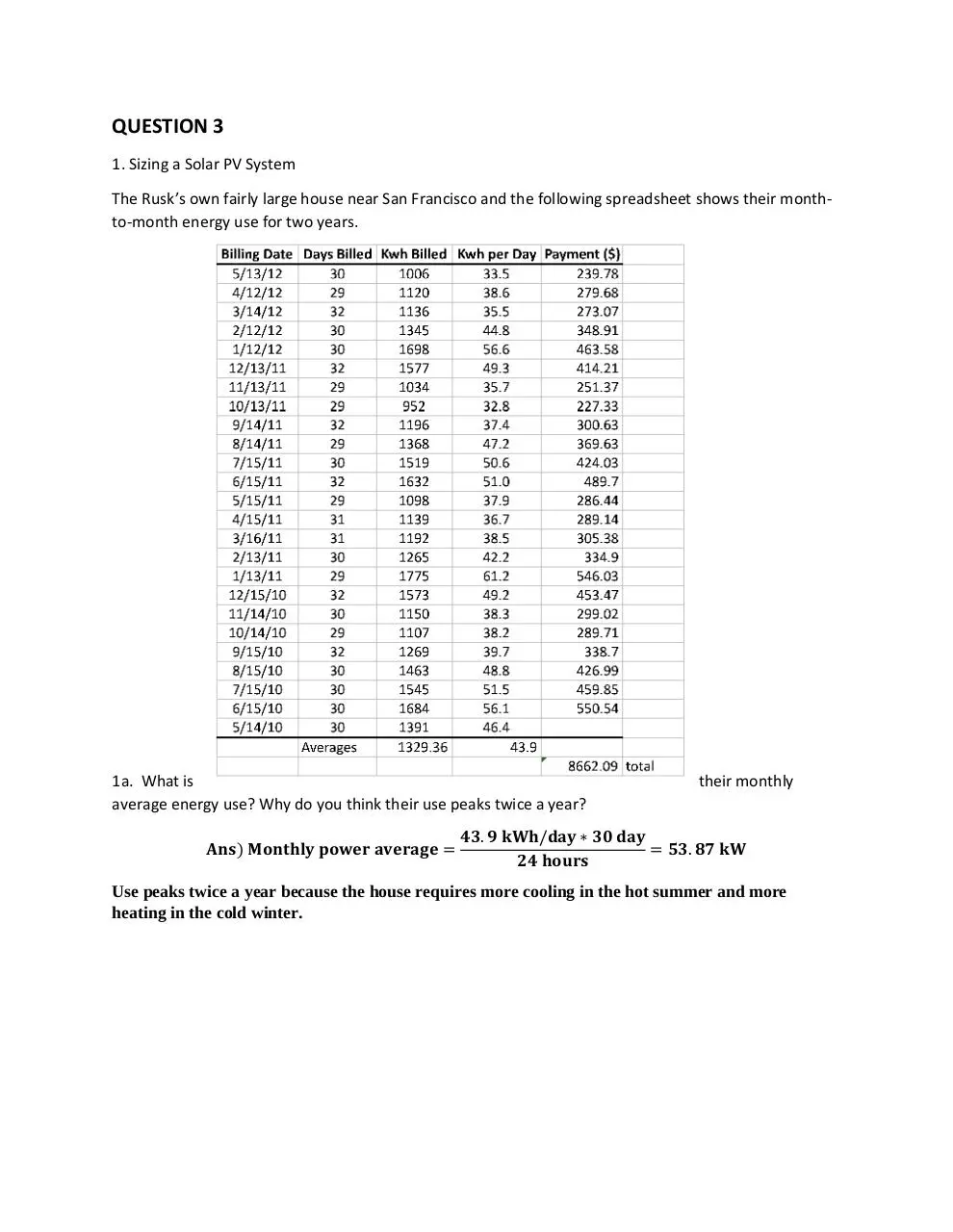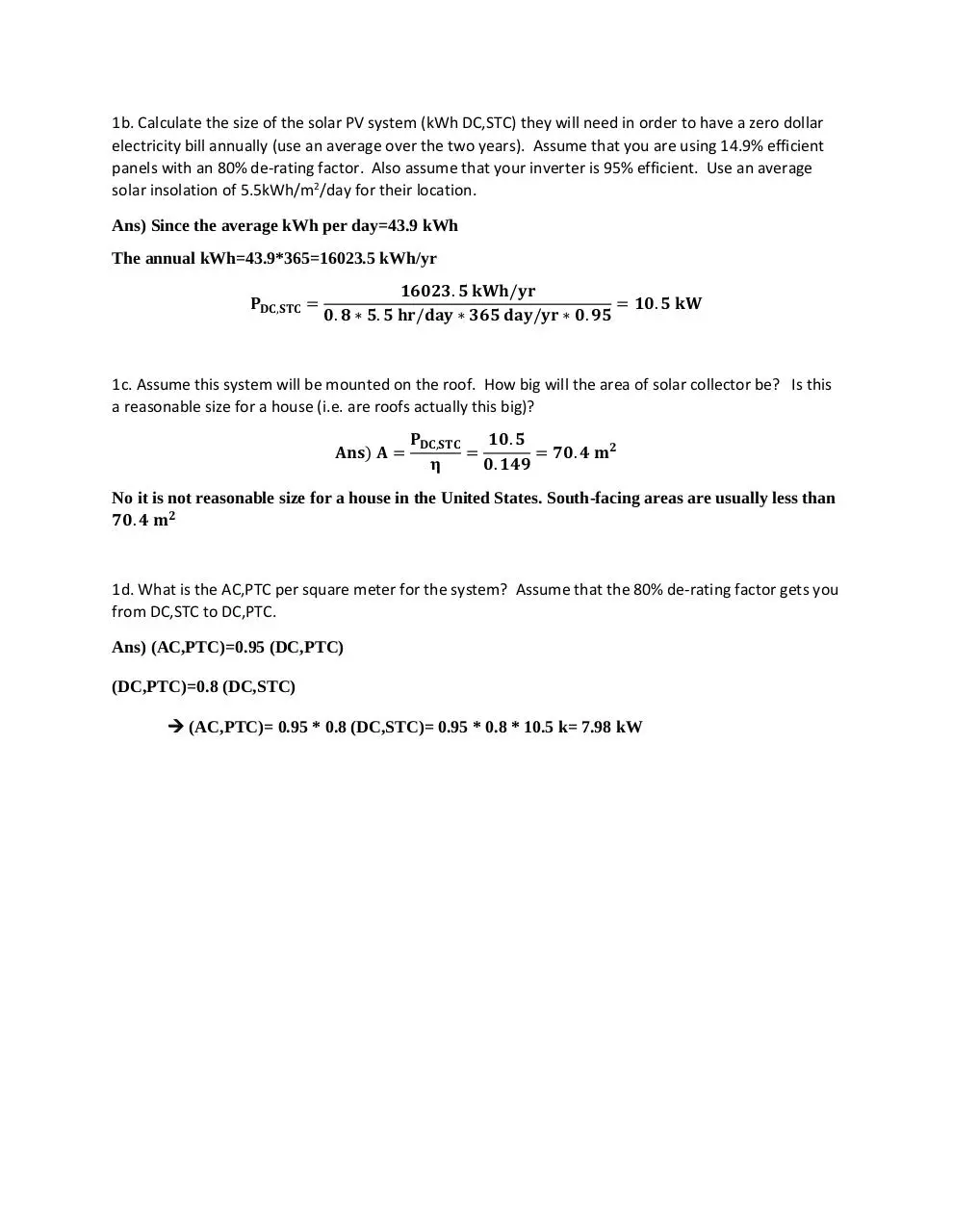ELEN 280 - Final Exam - Winter 2017.pdf (PDF, 809.44 KB)

### Share on social networks

#### HTML Code

Copy the following HTML code to share your document on a Website or Blog